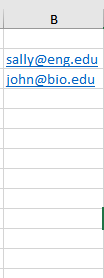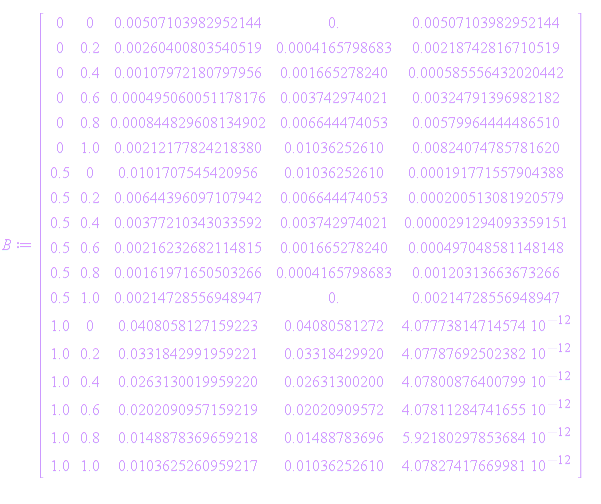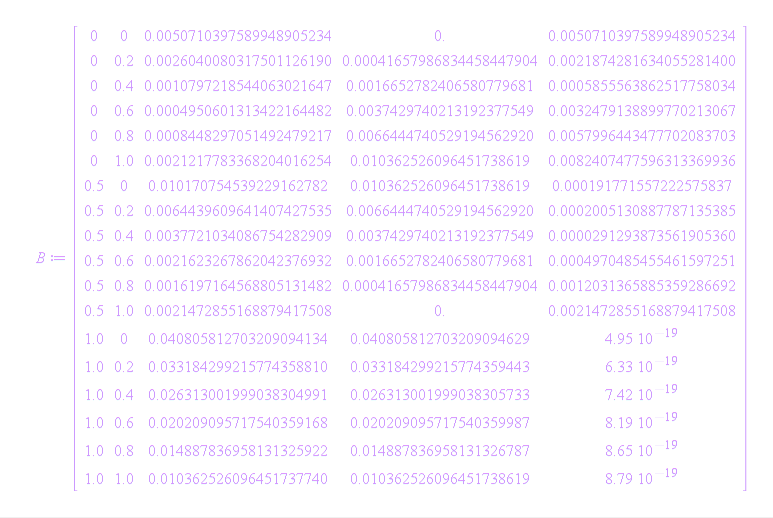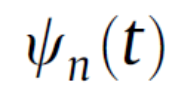5 years, 61 days

Getting data from 2D plots and 3Dplots ...

Maple 2020

I want to get data from 2D plots and 3Dplots and want to export to Excel or Matlab.

For  2D plots, the following code works. The data can be exported to Excel. No problem.

restart:

P1:=plot(sin(x));
data1:=op([1,1],P1);

ExcelTools:-Export(data1, "C:/Users/student/Desktop/XLdat.csv", "Sheet1", "A1");
ExportMatrix("C:/Users/student/Desktop/XLdat.csv", data1);

1. But the following code doesn' t work for exporting the data to Matlab. Why?

restart: P1:=plot(sin(x));
dataMat:=plottools:-getdata(P1);
dataMat2:=convert(dataMat,Matrix):
ExportMatrix( "test.mat",dataMat2, target=Matlab);

2. How to write a code in order to get data from the 3D plot and to export to Excel or Matlab?

For example;

restart:
z=sin(x)*cos(y);
P2:=plot3d(z);

Maple 2020.2

Read an excel data and doing some operat...

Maple 2020

Suppose that we have a excel file as follows:We can import the excel file to Maple as follows:

restart:
ExcelTools:-Import("C:\\Users\\student\\list.xlsx", "Page1");
• QUESTION 1: How to filter the cell including ".edu" in the column A and how to print them to column B as follows:Maple

Dear there,

I have a code.mw

• If I don't use command Digits in the code, I get the following result:Question 1: The third column of matrix B includes 16 decimal, but the fourth column of matrix B includes 11 decimal. Why?

• When I write the command Digits:=20, I get the following result:Question 2: The last column of matrix B shows the absolute error of the method.

When asked what is the absolute error of the method, what is the right answer?  (Because the higher the digit is chosen, the lower the absolute error is.)

Question 3: Are the two results consistent? How can we explain to someone who asks why the difference comes from?

Finding the integral of a piecewise func...

Maple 2020

The interval [0,T] is divided into n subintervals [i*h, (i + 1)*h], i = 0,1,2, . . . ,n -1, of equal lengths h where h=T/n.

The first (n + 1) hat functionsis defined as follows: